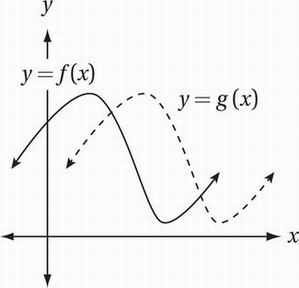# SAT Math Multiple Choice Question 749: Answer and Explanation

### Test Information

Question: 749

14.The figure above shows the graphs of functions f and g in the xy-plane. Which of the following equations could express the relationship between f and g?

• A. f(x) = g(x - 2)
• B. f(x) = g(x + 2)
• C. f(x) = g(x) + 2
• D. f(x) = g(x) - 2